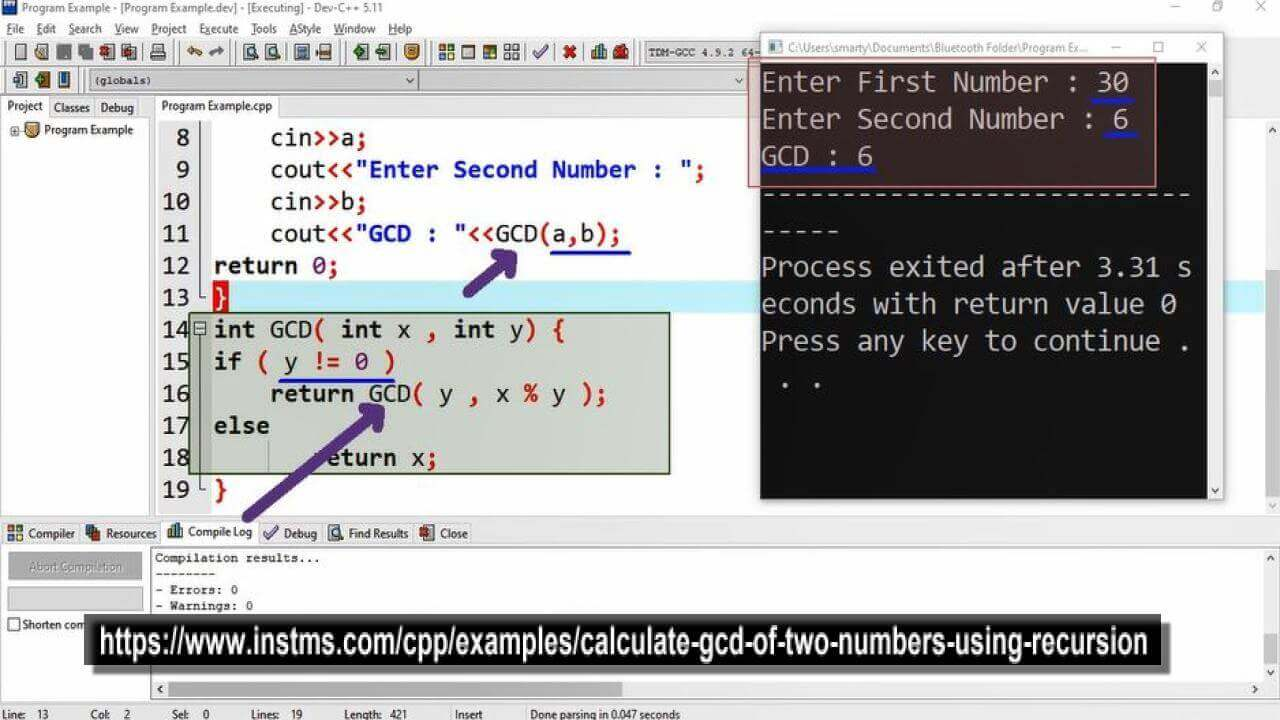# Calculate Gcd Of Two Numbers Using Recursion in CPP

## Source Code

#include <iostream>

using namespace std;

int GCD( int );

int main(int argc, char** argv) {

int a,b;  // variable declaration for input

// Taking input in variables
cout<<"Enter First Number : ";
cin>>a;

cout<<"Enter Second Number : ";
cin>>b;
cout<<"GCD : "<<GCD(a,b);

return 0;
}

int GCD( int x , int y) {

if ( y != 0 )
return GCD( y , x % y );

else
return x;
}

## Output## Working

Greatest common divisor is the common largest number that can divide the provide numbers. In this program example recursion is used for GCD calculation. This recursive call will get remainder of given two numbers until one of these equal to zero.